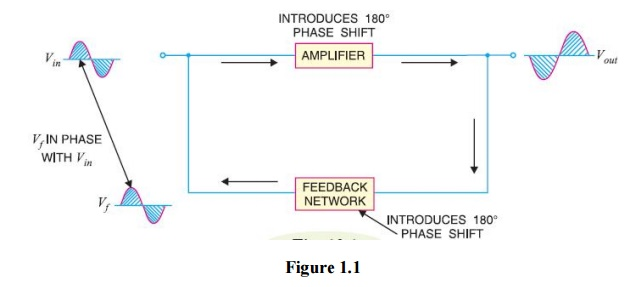# Feedback

The process of injecting a fraction of output energy of some device back to the input is known as feedback.

Feedback

The process of injecting a fraction of output energy of some device back to the input is known as feedback. The principle of feedback is probably as old as the invention of first machine but it is only some 50 years ago that feedback has come into use in connection with electronic circuits. It has been found very useful in reducing noise in amplifiers and making amplifier operation stable. Depending upon whether the feedback energy aids or opposes the input signal, there are two basic types of feedback in amplifiers viz positive feedback and negative feedback.

(i)          Positive feedback. When the feedback energy (voltage or current) is in phase with the input signal and thus aids it, it is called positive feedback. This is illustrated in Fig. 1.1. Both amplifier and feedback network introduce a phase shift of 180°. The result is a 360° phase shift around the loop, causing the feedback voltage Vf to be in phase with the input signal Vin.The positive feedback increases the gain of the amplifier. However, it has the disadvantages of increased distortion and instability. Therefore, positive feedback is seldom employed in amplifiers. One important use of positive feedback is in oscillators. As we shall see in the next chapter, if positive feedback is sufficiently large, it leads to oscillations. As a matter of fact, an oscillator is a device that converts d.c. power into a.c. power of any desired frequency.

(ii) Negative feedback. When the feedback energy (voltage or current) is out of phase with the input signal and thus opposes it, it is called negative feedback. This is illustrated in Fig. 1.2. As you can see, the amplifier introduces a phase shift of 180° into the circuit while the feedback network is so designed that it introduces no phase shift (i.e., 0° phase shift). The result is that the feedback voltage Vf is 180° out of phase with the input signal Vin.Negative feedback reduces the gain of the amplifier. However, the advantages of negative feedback are: reduction in distortion, stability in gain, increased bandwidth and improved input and output impedances. It is due to these advantages that negative feedback is frequently employed in amplifiers.

Study Material, Lecturing Notes, Assignment, Reference, Wiki description explanation, brief detail
Electronic Circuits : Feed Back Amplifiers : Feedback |